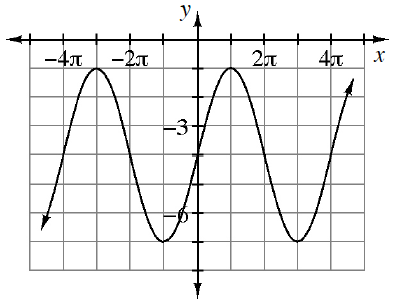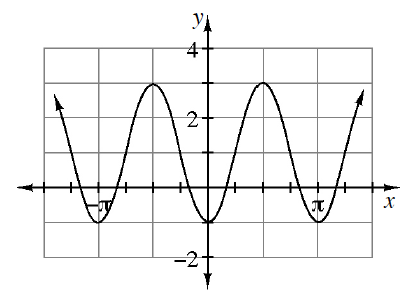### Home > PC3 > Chapter 12 > Lesson 12.1.2 > Problem12-32

12-32.

For each graph below, write two equations, one that uses sine and one that uses cosine, that will generate the graph.

1.The sine function does not need a horizontal shift.
The cosine function needs to be shifted $π$ units left.

1.The sine needs to be shifted $\frac{\pi}{4}$ units left.

The cosine needs to be reflected vertically.